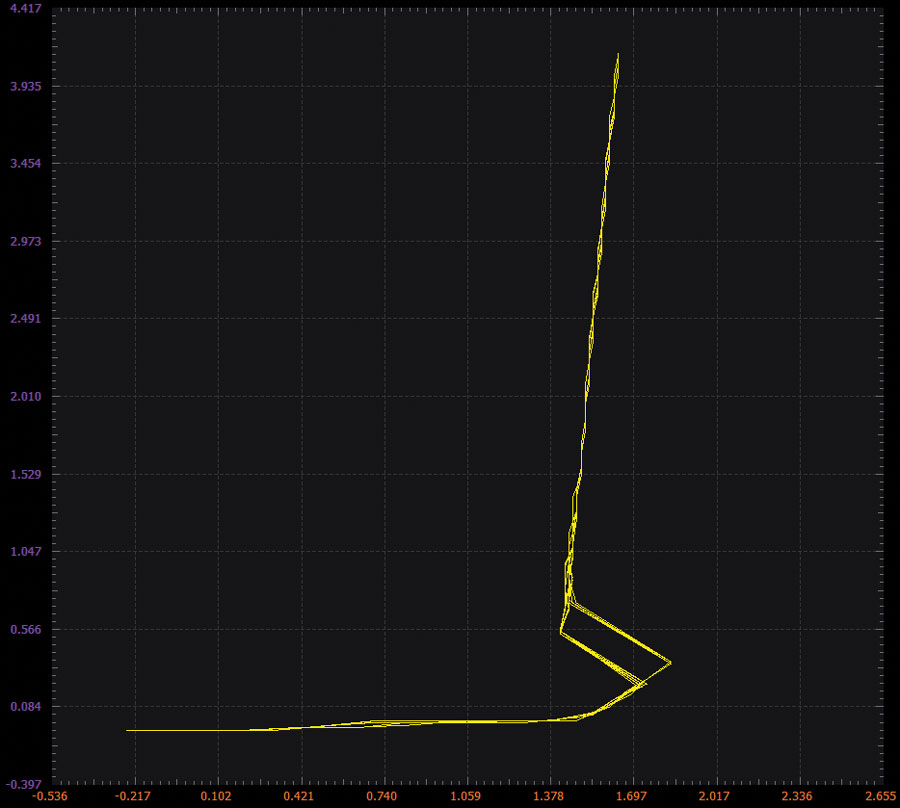# ADALM2000 Activity: A Floating (2-Terminal) Current Source/Sink

### Objective

The objective of this activity is to investigate the ΔVBE concept to produce an output current that is stabilized (less sensitive) to variations of the input voltage level. Feedback is used to build a circuit that produces a constant or regulated output current over a range of supply voltages.

#### Materials

• ADALM2000 Active Learning Module
• One 500 Ω variable resistor, potentiometer
• One 100 Ω resistor
• Three small signal NPN transistors (2N3904)
• Three small signal PNP transistors (2N3906)

#### Directions

Build the circuit shown in Figure 1 on your solderless breadboard. The blue boxes indicate where to connect the ADALM2000. PNP transistors Q1, Q2, and Q3 form a current mirror with a gain of 2; the output current is twice the input current. NPN transistors Q4, Q5, and Q6 along with variable resistor R1 form the ΔVBE part of the circuit. Resistor R2 is used to measure the current flowing in the circuit (Scope Channel 2) as the voltage across the circuit changes (Scope Channel 1).Figure 1. Floating current source (as a sink tied to a negative supply).

The output current is set by R1. The difference in VBE (ΔVBE) between Q4 and the parallel combination of Q5 and Q6 appears across R1. The PNP mirror—Q1, Q2, and Q3—has a gain of 2, if we assume they are of identical size. Thus, the current in Q4 is twice the combined current of Q5 and Q6. Again, if we assume Q4, Q5, and Q6 are also identical in size, the current density ratio is 4, and the difference in VBE will be:

Because of the absolute temperature term in this equation, the current will be proportional to absolute temperature. This can be a useful characteristic in certain instances but undesirable in others.

#### Hardware Setup

Breadboard connections for the circuit are presented in Figure 2.Figure 2. Floating current source (as a sink tied to a negative supply) breadboard circuit.

#### Procedure

Configure waveform generator W1 as a triangle wave with a frequency of 100 Hz and an amplitude of 10 V p-p with 0 V offset. The scope display should be set in both voltage vs. time and in XY mode with Channel 1 on the horizontal axis and Channel 2 on the vertical axis. Be sure to turn on the power supply only after you have completed and double checked your connections.Figure 3. Floating current source (as a sink tied to a negative supply) Scopy XY plot example.Figure 4. Floating current source (as a sink tied to a negative supply) LTspice XY plot example using ideal components.

### Prove the Floating Nature of the Circuit

In Figure 1 we referenced the negative end of the circuit to a negative power supply. To prove that this circuit is truly a floating current source, rearrange your breadboard to look like Figure 5 and repeat your measurements.Figure 5. Floating current source (as a source tied to a positive supply).

#### Hardware Setup

Breadboard connections for the circuit are presented in Figure 6.Figure 6. Floating current source (as a sink tied to a positive supply) breadboard circuit.

#### Procedure

Configure waveform generator W1 as a triangle wave with a frequency of 100 Hz and an amplitude of 10 V p-p with 0 V offset. The scope display should be set in both voltage vs. time and in XY mode with Channel 1 on the horizontal axis and Channel 2 on the vertical axis. Be sure to turn on the power supply only after you have completed and double checked your connections.Figure 7. Floating current source (as a sink tied to a positive supply) XY plot.Figure 8. Floating current source (as a sink tied to a positive supply) LTspice XY plot example using ideal components.

### Question:

What is the minimum voltage that the current source needs across it to maintain a more or less constant current, by analyzing the LTspice® plots for the circuits?

You can find the answer at the StudentZone blog.